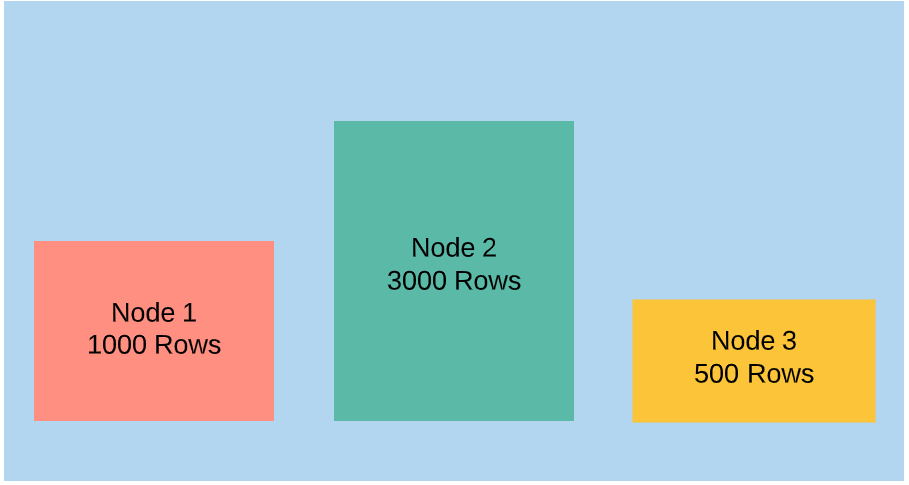# Tables with High Skew

## RStoolKit Result: Tables with high skew

If we are going to use a table with Dist Key style distribution, and you choose a wrong column, then you can get this problem. This may lead to more disk usage on a particular node and high CPU consumption on the node where the table has more number of rows.

See the below image, if we have table with wrong distribution style, then you can see the data skew. If you are running a query to fetch the data, Node1,Node3 will be complete the execution in very shot time, but the Node2 need more time to complete the process.## How the row skew is calculated in RedShift:

``````Most rows on a node / fewer rows on a node
``````

Lets calculate this for the above image.

``````3000/500 = 6
``````

There is fixed value for the health check. because its depends on the row count. If it is 100 then the table has no skew and you have the right dist key.

## Find the tables with High Skew:

``````select
"schema",
"table",
skew_rows
FROM
svv_table_info
WHERE
diststyle LIKE 'KEY%'
AND skew_rows > 3
AND "schema" not like 'pg_temp%';
``````

## Find the row count of all tables by Node wise.

``````select
a."name" ,
b.node,
sum(a.num_values)
from
pg_catalog.svv_diskusage a
join stv_slices b on
a.slice = b.slice
-- where a."name" = 'table_name'
group by
a."name",
b.node
order by
b.node
``````

## Find the row count of a table by Slice wise

``````select
slice,
sum(num_values)
from
pg_catalog.svv_diskusage
where
name = 'table_name'
group by
slice
order by
slice
``````

## How to fix this problem:

Its a fundamental table design problem. You have chose the column for the distkey very carefully. Better review your table and see whether do you need the distribution style as key or some other method.

· aws, redshift, rstoolkit